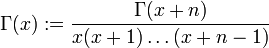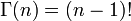# Gamma function

This article is about a particular function from a subset of the real numbers to the real numbers. Information about the function, including its domain, range, and key data relating to graphing, differentiation, and integration, is presented in the article.
View a complete list of particular functions on this wiki

## Definition

The gamma function is a function defined for all reals except zero and the negative integers. For positive reals, it is defined as:$\Gamma(x) := \int_0^\infty e^{-t}t^{x - 1} \, dt$

If$x$ is not positive and not an integer, define$\Gamma(x)$ as follows: pick a natural number$n$ such that$x + n$ is positive. Then:$\Gamma(x) := \frac{\Gamma(x +n)}{x(x+1)\dots (x + n - 1)}$

For$n$ a positive integer, it is true that$\Gamma(n) = (n - 1)!$.